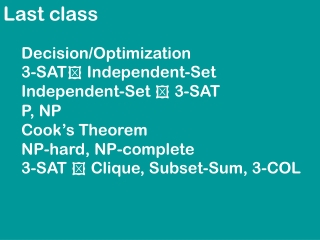DownloadDownload PresentationLast class

# Last class

Télécharger la présentation## Last class

- - - - - - - - - - - - - - - - - - - - - - - - - - - E N D - - - - - - - - - - - - - - - - - - - - - - - - - - -
##### Presentation Transcript

1. Last class Decision/Optimization 3-SAT Independent-Set Independent-Set  3-SAT P, NP Cook’s Theorem NP-hard, NP-complete 3-SAT  Clique, Subset-Sum, 3-COL

2. Reductions A  B all reductions we had were: INSTANCE of A  INSTANCE of B (many-to-one reductions) the black-box intuition model allowed more questions to an oracle for B (Turing reductions)

3. Planar-3-COL INSTANCE: planar graph G QUESTION: can the vertices of G be assigned colors red,green,blue so that no two neighboring vertices have the same color?

4. 3-COL  Planar-3-COL

5. 4-COL INSTANCE: graph G QUESTION: can the vertices of G be assigned one of 4 colors so that no two neighboring vertices have the same color?

6. 3-COL  4-COL  G G

7. planar 4-COL INSTANCE: planar graph G QUESTION: can the vertices of G be assigned one of 4 colors so that no two neighboring vertices have the same color?

8. planar 3-COL  planar 4-COL ?

9. planar 3-COL  planar 4-COL ? planar 4-COL is very easy: the answer is always yes. (4-color theorem, Appel, Haken)

10. Integer linear-programming INSTANCE: variables x1,...,xn collection of linear inequalities over the xi with integer coefficients QUESTION: does there exist an assignment of integers to the xi such that all the linear inequalities are satisfied?

11. Integer linear-programming INSTANCE: variables x1,...,xn collection of linear inequalities over the xi with integer coefficients QUESTION: does there exist an assignment of integers to the xi such that all the linear inequalities are satisfied? x1  1 x2  16 x1 x3  16 x2 x4  16 x3 x3+x4+x1  10000

12. Integer linear-programming we will show that ILP is NP-hard by showing 3-SAT  ILP true = 1, false =0 y1 y2 y3  x1 + (1-x2) + x3 1 0 x1 1 .... 0 xn 1

13. Integer linear-programming Is integer linear programming NP-complete ? I.e., is ILP in NP ? Witness of solvability = solution, but a priori we do not know that the solution is polynomially bounded. ILP NP, but the proof is far from trivial.

14. Min-Cut problem cut S  V number of edges crossing the cut | { {u,v} ; u S, v V-S } | INPUT: graph G OUTPUT: cut S with the minimum number of crossing edges

15. Min-Cut problem in P for each s,t pair run max-flow algorithm

16. Max-Cut problem cut S  V number of edges crossing the cut | { {u,v} ; u S, v V-S } | INPUT: graph G OUTPUT: cut S with the maximum number of crossing edges

17. Max-Cut problem INSTANCE: graph G, integer K QUESTION: does G have a cut with  K crossing edges?

18. Max-Cut problem INSTANCE: graph G, integer K QUESTION: does G have a cut with  K crossing edges? NAE-3-SAT  Max-Cut NAE-3-SAT INSTANCE: 3-CNF formula QUESTION: does there exist an assignment such that every claues have  1 false and  1 true ?

19. NAE-3-SAT  Max-Cut INSTANCE: 3-CNF formula QUESTION: does there exist an assignment such that every claues have  1 false and  1 true ? 1 vertex for each literal x1 x2 x3 x3 x2 2m parallel edges x1 x2

20. 3-SAT  NAE-3-SAT y1 y2 y3  y1 y2 zi , zi y3 b • C satisfiable  can find 3-NAE assignment for C’ • C’ has 3-NAE assignment  C satisfiable

21. Is NPco-NP = P ? Factoring INPUT: integer n OUTPUT: factorization of n, i.e., n=p11 ... pkk Factoring – decision version INSTANCE: pair of integers n,k QUESTION: does n have a factor x{2,...k} ?